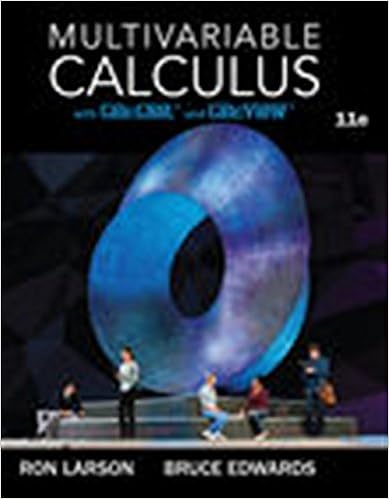# 7 the population of the world was about 53 billion in

• Notes
• mimi304
• 56
• 50% (2) 1 out of 2 people found this document helpful

This preview shows page 35 - 37 out of 56 pages.

##### We have textbook solutions for you!
The document you are viewing contains questions related to this textbook.The document you are viewing contains questions related to this textbook.
Chapter 16 / Exercise 15
Multivariable Calculus
LarsonExpert Verified
7. The population of the world was about 5.3 billion in 1990. Birth rates in the 1990s ranged from 35 to 40 million per year and death rates ranged from 15 to 20 million per year. Let’s assume that the carrying capacity for world population is 100 billion. (a) Write the logistic differential equation for these data. (Because the initial population is small compared to the y t t M 8 10 7 kg k 0.71 y 0 2 10 7 kg 4 10 7 kg P t dP dt 0.4 P 0.001 P 2 P 0 50 t P 0 9.4 Exercises ; Graphing calculator or computer required Computer algebra system required 1. Homework Hints available at stewartcalculus.com CAS Time (hours) Yeast cells Time (hours) Yeast cells 0 18 10 509 2 39 12 597 4 80 14 640 6 171 16 664 8 336 18 672
##### We have textbook solutions for you!
The document you are viewing contains questions related to this textbook.The document you are viewing contains questions related to this textbook.
Chapter 16 / Exercise 15
Multivariable Calculus
LarsonExpert Verified
638 CHAPTER 9 carrying capacity, you can take to be an estimate of the initial relative growth rate.) (b) Use the logistic model to estimate the world population in the year 2000 and compare with the actual population of 6.1 billion. (c) Use the logistic model to predict the world population in the years 2100 and 2500. (d) What are your predictions if the carrying capacity is 50 billion? 8. (a) Make a guess as to the carrying capacity for the US population. Use it and the fact that the population was 250 million in 1990 to formulate a logistic model for the US population. (b) Determine the value of in your model by using the fact that the population in 2000 was 275 million. (c) Use your model to predict the US population in the years 2100 and 2200. (d) Use your model to predict the year in which the US population will exceed 350 million. 9. One model for the spread of a rumor is that the rate of spread is proportional to the product of the fraction of the popula- tion who have heard the rumor and the fraction who have not heard the rumor. (a) Write a differential equation that is satisfied by . (b) Solve the differential equation. (c) A small town has 1000 inhabitants. At 8 AM , 80 people have heard a rumor. By noon half the town has heard it. At what time will of the population have heard the rumor? 10. Biologists stocked a lake with 400 fish and estimated the carrying capacity (the maximal population for the fish of that species in that lake) to be 10,000. The number of fish tripled in the first year. (a) Assuming that the size of the fish population satisfies thelogistic equation, find an expression for the size of thepopulation after years. (b) How long will it take for the population to increase to 5000? 11. (a) Show that if satisfies the logistic equation , then (b) Deduce that a population grows fastest when it reaches half its carrying capacity. ;
•••